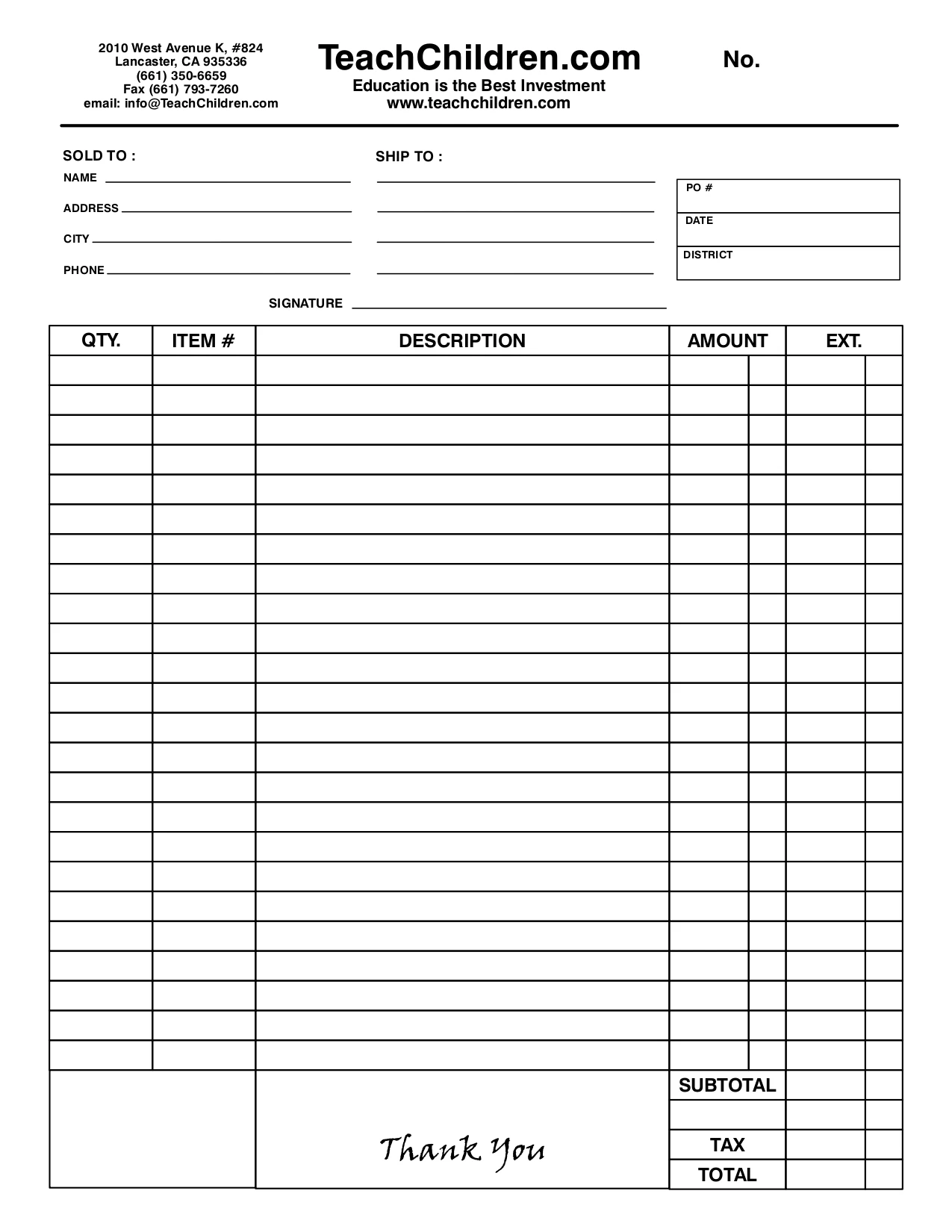×# Daily Word Problems Math, Grade 6

(No reviews yet)
SKU:
EMC3096
UPC:
9.78E+12
\$36.36

## Description

Completely revised in 2019 to reflect grade-level standards, Daily Word Problems is the perfect resource to improve students' problem-solving skills. The word problems are written to support current math standards and expectations and provide consistent spiral review of math concepts. Students' problem-solving skills improve as they participate in meaningful, real-life math practice. The 36 weeks of activities provide practice of grade-level math concepts such as addition, multiplication, fractions, logic, algebra, and more. Each week focuses on a theme and provides a daily word problem within the context of a real-life situation. • Monday–Thursday's activities provide a one- or two-step word problem. • Friday's format is more extensive and requires multiple steps. The multi-step problems require students to incorporate higher-order thinking skills by applying their understanding within a different context. • Reproducible pages provide plenty of room for students to solve using the strategy of their choice. What's new in the revised edition of Daily Word Problems? • All-new word problems to support current standards. • New weekly themes that present problems in the context of real life • Day-by-day skills list to help teachers identify skills practiced Grade 6 math skill practice includes: • positive and negative numbers • addition and subtraction • multiplication and division • factors and multiples • fractions • decimals • percentages • ratios and rates • inequalities • time and temperature • linear measurement • weight and capacity • perimeter and area • surface area and volume • statistics • coordinate plane • graphs, charts, and maps • logical thinking • spatial reasoning • expressions and equations Includes scope and sequence chart and an answer key.

Key Features :

i : Develop students' problem solving with Daily Word Problems
ii : The 36 weeks of word problems provide grade-level math practice of important concepts such as addition, multiplication, fractions, logic, algebra, and more
iii : Weekly themed word problems present math within the context of real-world situations.
iv : Lessons provide spiral review of math concepts and problem solving.
v : 288 pages
View AllClose
×